Courses

# Classical and Quantum Statistics (Part - 1) - CSIR-NET Physical Sciences Physics Notes | EduRev

## Physics for IIT JAM, UGC - NET, CSIR NET

Created by: Akhilesh Thakur

## Physics : Classical and Quantum Statistics (Part - 1) - CSIR-NET Physical Sciences Physics Notes | EduRev

The document Classical and Quantum Statistics (Part - 1) - CSIR-NET Physical Sciences Physics Notes | EduRev is a part of the Physics Course Physics for IIT JAM, UGC - NET, CSIR NET.
All you need of Physics at this link: Physics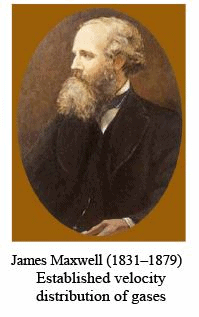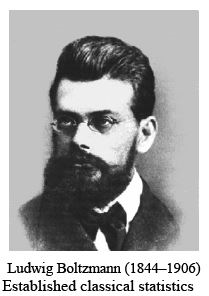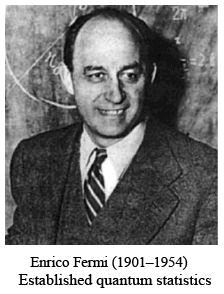Classical and quantum statistics

Classical Maxwell–Boltzmann statistics and quantum mechanical Fermi–Dirac statistics are introduced to calculate the occupancy of states. Special attention is given to analytic approximations of the Fermi–Dirac integral and to its approximate solutions in the nondegenerate and the highly degenerate regime. In addition, some numerical approximations to the Fermi–Dirac integral are summarized.

Semiconductor statistics includes both classical statistics and quantum statistics. Classical or Maxwell–Boltzmann statistics is derived on the basis of purely classical physics arguments. In contrast, quantum statistics takes into account two results of quantum mechanics, namely (i) the Pauli exclusion principle which limits the number of electrons occupying a state of energy E and (ii) the finiteness of the number of states in an energy interval E and E + dE. The finiteness of states is a result of the Schrödinger equation. In this section, the basic concepts of classical statistics and quantum statistics are derived. The fundamentals of ideal gases and statistical distributions are summarized as well since they are the basis of semiconductor statistics.

Probability and distribution functions

Consider a large number N of free classical particles such as atoms, molecules or electrons which are kept at a constant temperature T, and which interact only weakly with one another. The energy of a single particle consists of kinetic energy due to translatory motion and an internal energy for example due to rotations, vibrations, or orbital motions of the particle. In the following we consider particles with only kinetic energy due to translatory motion. The particles of the system can assume an energy E, where E can be either a discrete or a continuous variable. If Ni particles out of N particles have an energy between Ei and E+ dE, the probability of any particle having any energy within the interval Ei and E+ dE is given by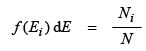(13.1)

where f(E) is the energy distribution function of a particle system. In statistics, f(E) is frequently called the probability density function. The total number of particles is given by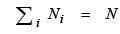(13.2)

where the sum is over all possible energy intervals. Thus, the integral over the energy distribution function is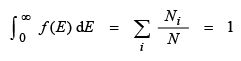(13.3)

In other words, the probability of any particle having an energy between zero and infinity is unity. Distribution functions which obey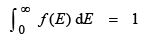(13.4)

are called normalized distribution functions. The average energy or mean energy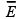of a single particle is obtained by calculating the total energy and dividing by the number of particles, that is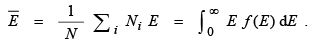(13.5)
In addition to energy distribution functions, velocity distribution functions are valuable. Since only the kinetic translatory motion (no rotational motion) is considered, the velocity and energy are related by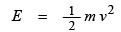(13.6)
The average velocity and the average energy are related by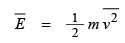(13.7)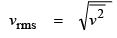(13.8)

and is the velocity corresponding to the average energy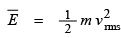(13.9)

In analogy to the energy distribution we assume that Ni particles have a velocity within the interval vi and v+ dv. Thus,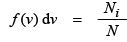(13.10)

where f(v) is the normalized velocity distribution. Knowing f(v), the following relations allow one to calculate the mean velocity, the mean square velocity, and the root-mean-square velocity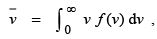(13.11)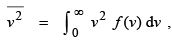(13.12)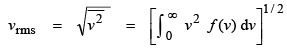(13.13)

Up to now we have considered the velocity as a scalar. A more specific description of the velocity distribution is obtained by considering each component of the velocity v = (vx, vy, vz). If Ni particles out of N particles have a velocity in the ‘volume’ element v+ dvx, vy + dvy, and v+ dvz, the distribution function is given by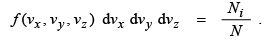(13.14)

Since ΣNi  =  N, the velocity distribution function is normalized, i. e.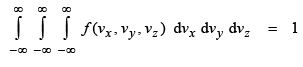(13.15)

The average of a specific propagation direction, for example vx is evaluated in analogy to Eqs. (13.11 – 13). One obtains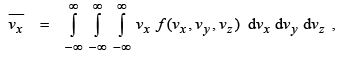(13.16)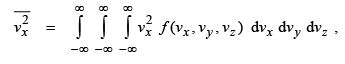(13.17)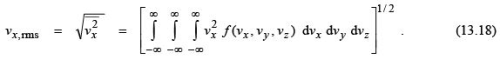In a closed system the mean velocities are zero, that is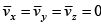. However, the mean square velocities are, just as the energy, not equal to zero.

Ideal gases of atoms and electrons

The basis of classical semiconductor statistics is ideal gas theory. It is therefore necessary to make a small excursion into this theory. The individual particles in such ideal gases are assumed to interact weakly, that is collisions between atoms or molecules are a relatively seldom event. It is further assumed that there is no interaction between the particles of the gas (such as electrostatic interaction), unless the particles collide. The collisions are assumed to be (i) elastic (i. e. total energy and momentum of the two particles involved in a collision are preserved) and (ii) of very short duration.

Ideal gases follow the universal gas equation

P V   =   R T (13.19)
where P is the pressure, V the volume of the gas, T its temperature, and R is the universal gas constant. This constant is independent of the species of the gas particles and has a value of R = 8.314 J K–1 mol–1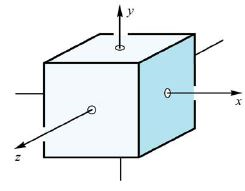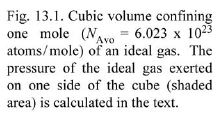Next, the pressure P and the kinetic energy of an individual particle of the gas will be calculated. For the calculation it is assumed that the gas is confined to a cube of volume V, as shown in Fig. 13.1. The quantity of the gas is assumed to be 1 mole, that is the number of atoms or molecules is given by Avogadro’s number, NAvo = 6.023 × 1023 particles per mole. Each side of the cube is assumed to have an area A = V 2/3. If a particle of mass m and momentum m v(along the x-direction) is elastically reflected from the wall, it provides a momentum 2 m vx  to reverse the particle momentum. If the duration of the collision with the wall is dt, then the force acting on the wall during the time dt is given by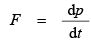(13.20)
where the momentum change is dp = 2 m vx. The pressure P on the wall during the collision with one particle is given by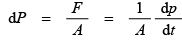(13.21)
where A is the area of the cube’s walls. Next we calculate the total pressure P experienced by the wall if a number of NAvo particles are within the volume V. For this purpose we first determine the number of collisions with the wall during the time dt. If the particles have a velocity vx, then the number of particles hitting the wall during dt is (NAvo / V) A vx dt. The fraction of particles

having a velocity vx is obtained from the velocity distribution function and is given by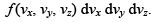. Consequently, the total pressure is obtained by integration over all positive velocities in the x-direction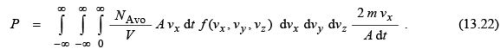Since the velocity distribution is symmetric with respect to positive and negative x-direction, the integration can be expanded from – ∞ to + ∞.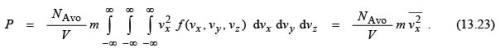Since the velocity distribution is isotropic, the mean square velocity is given by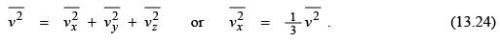The pressure on the wall is then given by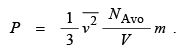(13.25)

Using the universal gas equation, Eq. (13.19), one obtains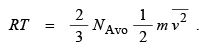(13.26)

The average kinetic energy of one mole of the ideal gas can then be written as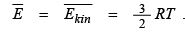(13.27)

The average kinetic energy of one single particle is obtained by division by the number of particles, i. e.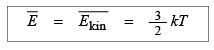(13.28)

where k = R / NAvo is the Boltzmann constant. The preceding calculation has been carried out for a three-dimensional space. In a one-dimensional space (one degree of freedom), the average velocity is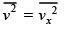and the resulting kinetic energy is given by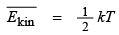(per degree of freedom) . (13.29)

Thus the kinetic energy of an atom or molecule is given by (1/2) kT. Equation (13.29) is called the equipartition law, which states that each ‘degree of freedom’ contributes (1/2) kT to the total kinetic energy.

159 docs

,

,

,

,

,

,

,

,

,

,

,

,

,

,

,

,

,

,

,

,

,

;